Our World Statistics Day conversations have been a great reminder of how much statistics can inform our lives. Do you have an example of how statistics has made a difference in your life? Share your story with the Community!
Choose Language Hide Translation Bar
Highlighted

## Not a JMP question, but hoping someone can help - Estimating StdDev from Min, Max, Avg

Firstly, this is not a JMP question but a gereral statistics question, so I apologize if it's inappropriate to post it here.  But I'm hoping someone in the JMP community can help with it.

I have a series of data samples, where each sample has Min, Avg (Mean), and Max recorded.  The data is sampled every second, but only recorded every 15 minutes.  So each of my 15-minute data points has the Min of the 1-second samples, the Max of the 1-second samples, and the Average of the 1-second samples over the 15-minute interval.

I have a number of therse 15-minute data points, say 24 hours worth (96 data points).  I'd like to try to estimate the StdDev of the value across the 24-hour period, as if I actually had all the 1-second samples over the 24-hour period.  I think if each of my 15-minute data points also had the StdDev of the 1-second samples this would be more straighforward, but I don't; just MIn, Max, and Avg.

Any guidance would be appreciated.

7 REPLIES 7
HighlightedjulianCommunity Manager

## Re: Not a JMP question, but hoping someone can help - Estimating StdDev from Min, Max, Avg

Hi @wsande,

I think you're in luck. With the data you have, you have no problem estimating the standard deviation of the variable at the original 1 sec sampling. All you need is the standard deviation (or variance) of the means themselves (the min and max are not needed).

Variances behave in predictable ways when operating on them. In this case, the operation between the original data, and the summarized data you have, is dividing by a constant (the number of observations, 900, the number of seconds in 15 min). The basis for the formula for the standard error is actually what we'll apply here. Recall that the standard deviation of the sampling distribution of the mean is Sigma / SqRoot(n), where sigma is the population standard deviation, and n is the sample size. In terms of variance, this is just variance/n -- pretty straightforward as far as formulas go!

So how does this help? We know you have 900 observations that go into each mean in your data table (60 samples/min * 15 min per recording). In the table you do have, you have of 96 observations (means for each 15 min, 4 per hour x 24 hours = 96). For illustration right now, suppose we know that the standard deviation of the means you have is 4. What standard deviation in the original 1-sec resolution would result in that if we had applied the formula sigma / sqroot(n)? Let's flip it around.

standard deviation of means = sigma/sqroot(n)

4 = sigma/sqroot(900)

4*sqroot(900) = sigma

sigma = 120.

Violla!

The distributional shape of the population doesn't matter. Variances work this way regardless of the underlying form. We don't have to trust the math, either. Let's do this up in JMP and see what happens. Here's a table of 86,400 observations, your 1sec resolution table: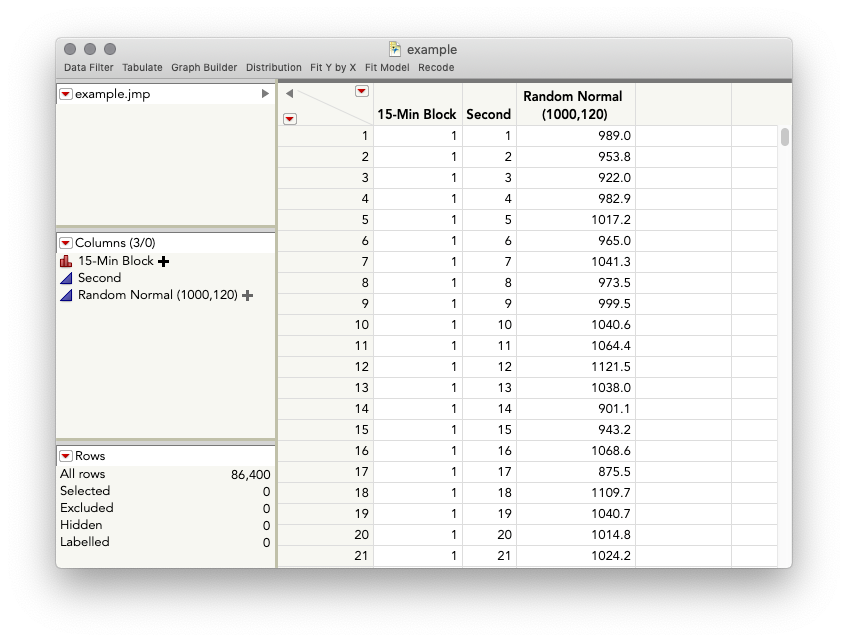This is the distribution of the original variable, made with a Random Normal() function: Mean of 1000, Standard deviation of 120 (well about, this is a random function, so it's close).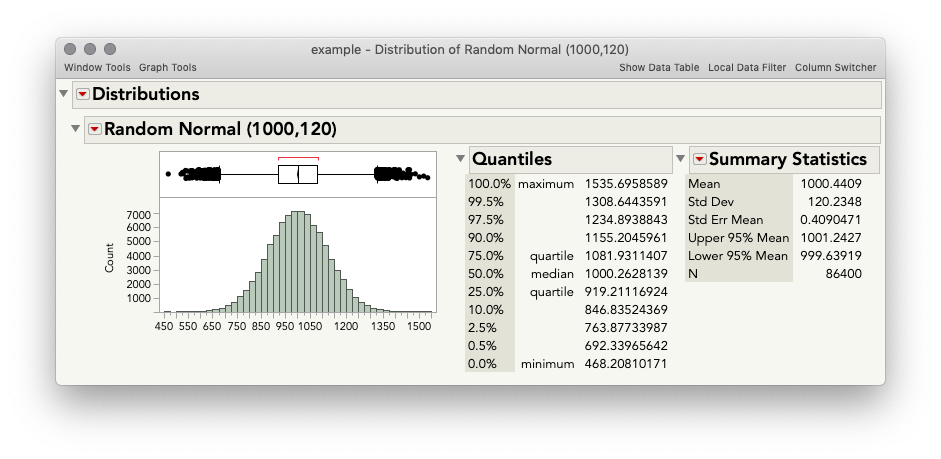Let's summarize by 15-min block using Tables > Summary: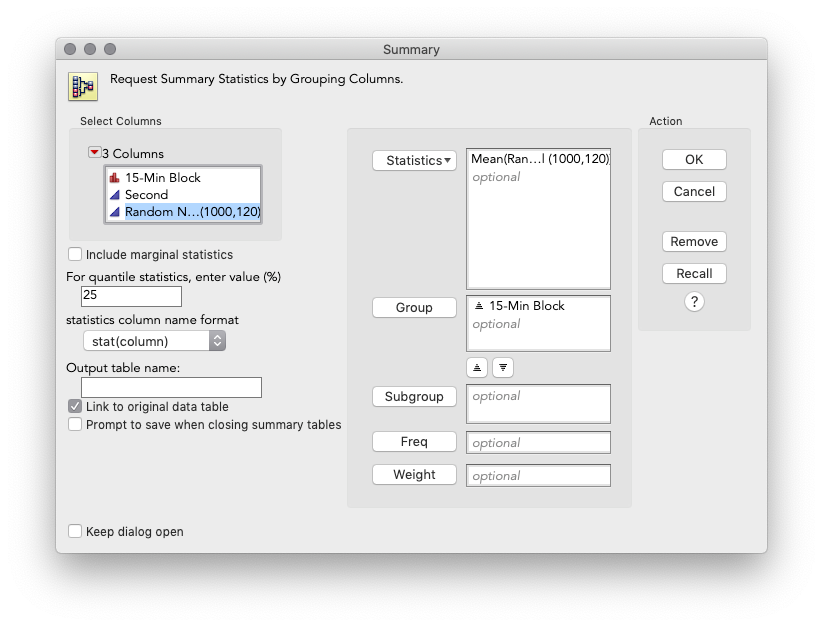This gives the summarized table below, with the mean for each block.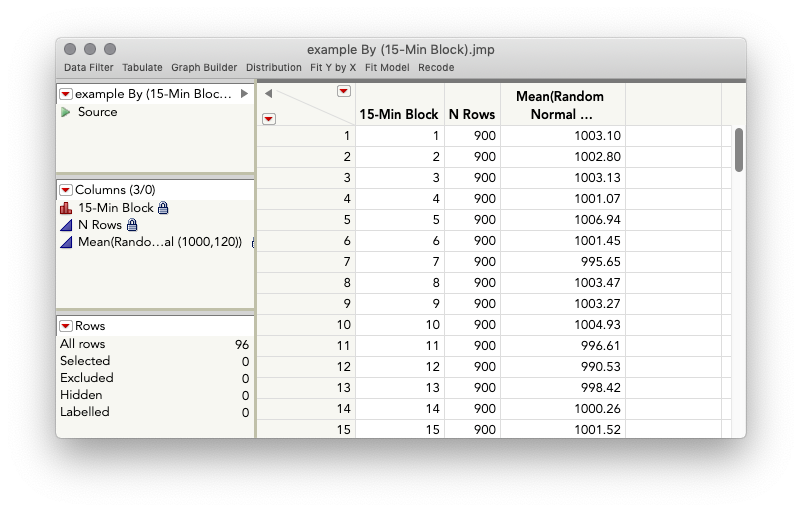Let's take a look at the distribution of the means (which is essentially what your final table is):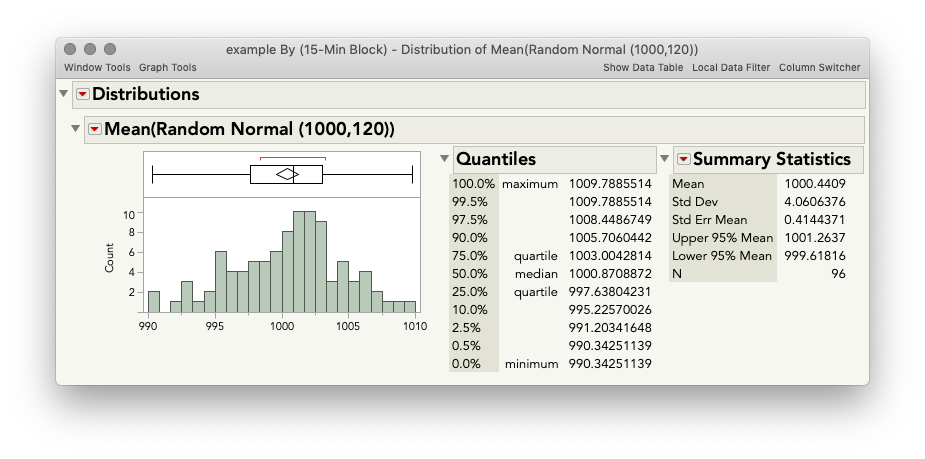And there we go, the standard deviation of the sampling distribution of the mean (which is what we've built) is 4 -- sigma / sqroot(n) just as theory tells us.

One caveat... this empirical sampling distribution is just 96 observations of the theoretically infinite sampling distribution, so every time you repeat this experiment you will get a slightly different estimate. If we were to build the original table to have millions and millions of 15-minute blocks and then sample thousands and thousands of means of those 15-minute blocks, we'd be pretty dead on. We could show this empirically, but we know it theoretically. And, the point really is that our best, unbiased estimate of the population sigma when we have the standard error (the standard deviation of the means), is to take that standard error and multiply it by sqroot(n).

So, TLDR: take the standard deviation you have of the means, and multiple by sqroot (900).

I hope this helps!

Highlighted

## Re: Not a JMP question, but hoping someone can help - Estimating StdDev from Min, Max, Avg

@julian 's response is a perfect answer if the underlying data is random with no strong systematic effects. The counter example below is extreme, but demonstrates how a systematic effect can result in a very stable mean, and hence, the std dev of the mean is an underestimate of the std error.

Using Julian's table, create a column called Systematic with the formula:

``````If(
Row() == 1, Random Integer( 850, 1150 ),
Modulo( Row(), 100 ) == 1, Random Integer( 850, 1150 ),
Modulo( Row(), 100 ) == 51, Lag( :Systematic, 1 ) * -1,
Lag( :Systematic, 1 )
)``````

Below is a screen shot of of the individual one second data for a selected 15-Min Block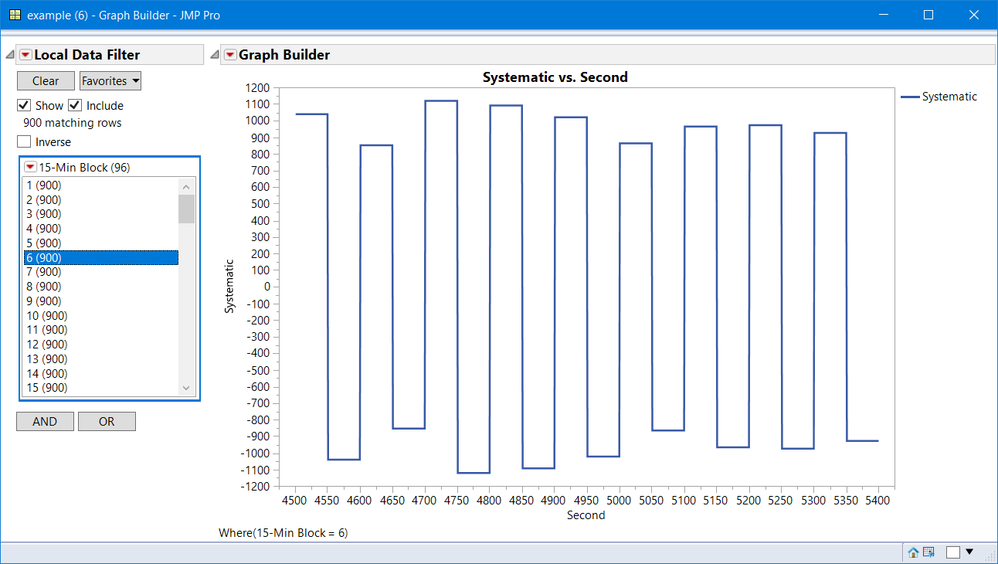You can see there is variation in the raw data. However, the summarized 15-Min Block data, all the means are zero, because this example is purposely set up to be an extreme case. Even if the systematic effects were not this extreme, a strong systematic pattern can make the mean artificially stable.

This counter example is not meant to undermine @julian 's solution, but meant to be a caution. If at all possible you should try to find a method to get to a couple random intervals of the raw data, to rule out strong systematic, cyclic events.

HighlightedjulianCommunity Manager

## Re: Not a JMP question, but hoping someone can help - Estimating StdDev from Min, Max, Avg

That it such an important point I neglected to mention, @gzmorgan0! I was assuming independent and identically distributed observations, but that certainly might not be the case.
Highlighted

## Re: Not a JMP question, but hoping someone can help - Estimating StdDev from Min, Max, Avg

To add some perspective to both @julian and @gzmorgan0 's replies, when I was sitting for the culminating oral exam in my MS in applied math and statistics program at Rochester Institute of Technology with the department head Dr. John Hromi and Professor Mason Wescott, I was asked this question first:

"Your boss hands you a pile of data that was collected over time and wants you to analyze it. What will you do next?" I think my reply went something like, "I'd create a histogram of the data and then, since the data is time series in nature either a run chart or control chart if I can create rational subgroups out of the data. Then I'd look for the middle, spread, anything unusual or supicious on the histogram and finally over on the run chart or control chart, stability in the mean and spread."

Of course I was a pile of nerves going into the exam. Two A list industrical statisticians who have forgotten more about statistics than I'll ever know.

Dr. Hromi smiled and said something like, "Good answer...let's proceed."

Highlighted

## Re: Not a JMP question, but hoping someone can help - Estimating StdDev from Min, Max, Avg

Thanks so much for the detailed explanation.  However, there is something going on with my data such that this is giving me results that don't quite make sense.

I have a data set from >80 hours, not just 24 hours.  So, > 300 samples.  The StdDev of the 15mAvg values is 0.00513.  With 900 1-second data ponts per 15-minute period, that would imply a population StdDev of 0.1538 (= 0.00513 * 30).  However the total range of my data over the 80+ hours is only 0.05 Max(15mMax) - Min(15mMin).  I would have expected the range to be several SD's not a fraction of an SD.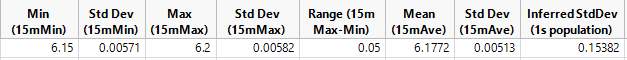I wonder if I'm running into a "quantization problem.  The resolution of my samples is 0.01, which is 2x the SD that I measuring on the sample averages.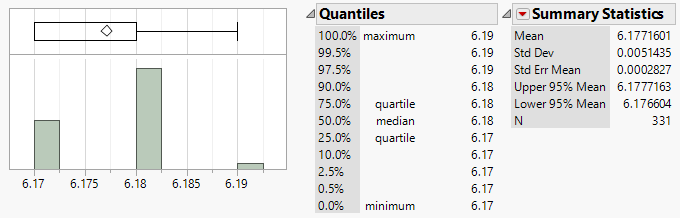I don't know if that's somehow skewing the results.

I actually have > 200 of these data sets, for different "channels", and typically the Range is 3x to 5x the StdDev of the sample means.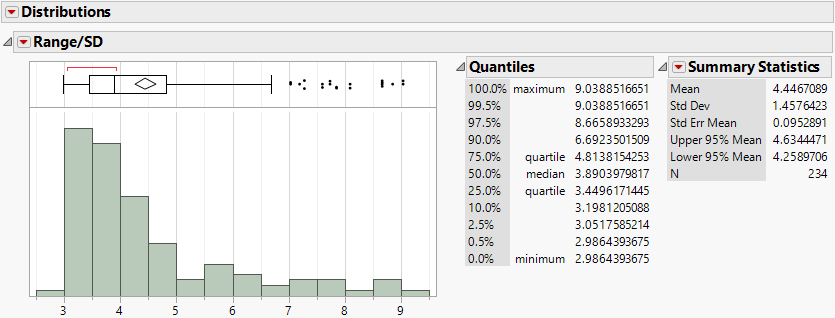One thing I'm wondering about...  If instead of 1-second samples, the underlying population was 10-second samples, then there would only be 90 samples per 15-minute interval, and the calculation of the population SD would change by a factor of 3.  ( SQRT(90) instead of SQRT(900) ).  Yet the underlying continuous process would not have changed.  I'm having a hard time wrapping my head around this...

Highlighted

## Re: Not a JMP question, but hoping someone can help - Estimating StdDev from Min, Max, Avg

1. From my experience with semiconductors, different channels can have different distributions, so just a caution with generalizing across multiple populations.
2. This suggestion aligns with @P_Bartell' s oral exam. I suggest for each channel you look at the distribution and a trend chart of  the 15 min ranges (15 min max - 15 min min).  Give the resolution of your data, I fear you might have many zeroes. Anyway long ago, when compute power and data storage were expensive, aggregated data was commonplace. A simple approach was to assume 95% of the sample's ranges would represent 4 std dev, that is, max = +2 std dev and min = -2 std dev, so range/4 was used as a std dev.  This might provide some insight into your data.
3. If #2's analysis has many zero ranges, this might be the reason, raw data is not reported, and 15 min intervals with ranges > 0, suggest a shift/change in the value. If this is the scenario, then the number of shifts/transitions per time period might be a better metric than std dev for the stability of the channel (or time between transistion).

I am not sure of your goal, but follow Peter's oral exam advice and plot the means and the ranges by sequence and look at the distributions.  Often summary statistics of time series data does not adequately describe the behavior of the unit being monitored.

JMP makes this easy to do with a couple clicks.

HighlightedjulianCommunity Manager

## Re: Not a JMP question, but hoping someone can help - Estimating StdDev from Min, Max, Avg

Hi @wsande,

I agree with @gzmorgan0 and @P_Bartell's suggestions, but let me tackle a few of your specific questions:

"The StdDev of the 15mAvg values is 0.00513... that would imply a population StdDev of 0.1538 (= 0.00513 * 30).  However the total range of my data over the 80+ hours is only 0.05 Max(15mMax) - Min(15mMin).  I would have expected the range to be several SD's not a fraction of an SD."

I can see how that might seem strange, but what you have is the range of the means of samples of size 900, not the range of the original data. if you look in my example before, the range of my means of size 900 was around 20. The range of the original data was over 1000. By collecting observations together and calculating a mean, the resulting numbers, each of them a mean of 900 observations, tend to be close to one another than the original data points are close to one another (and that's why we can state that the standard deviation of sample means will be smaller than the standard deviation of the original data by a factor of sqroot(n)). So, back to your case, the range of your means is 0.05, which given samples of 900 we know is substantially smaller than the range of the original data. Thus, it shouldn't be surprising that the standard deviation we estimate for the original generating data is larger.

"I wonder if I'm running into a "quantization problem.  The resolution of my samples is 0.01, which is 2x the SD that I measuring on the sample averages"

Given what we know from the sample means here, we should expect that the range of the data in the original 1sec samples is larger, so hopefully, you're not in a situation where your measurement device isn't capable of accurately measuring the phenomenon of interest.  I can't really speak to that, though.

"I don't know if that's somehow skewing the results.  I actually have > 200 of these data sets, for different "channels", and typically the Range is 3x to 5x the StdDev of the sample means."

I agree that we should expect that the range of the sample means is larger than the standard deviation of the sample means. But, that's even true in the first plot you showed, Range = 0.05, and StDev = 0.0051. However, with samples as large as 900, we shouldn't expect that the range of the sample means is larger than the standard deviation of the original variable from which the samples are drawn.

"One thing I'm wondering about...  If instead of 1-second samples, the underlying population was 10-second samples, then there would only be 90 samples per 15-minute interval, and the calculation of the population SD would change by a factor of 3.  ( SQRT(90) instead of SQRT(900) ).  Yet the underlying continuous process would not have changed.  I'm having a hard time wrapping my head around this..."

Fantastic question! It seems perplexing at first, but let me hopefully explain this another way. If in the original data you had data collected every 10 seconds, rather than every 1 second, the sample means in your final table would be built out of samples of size 90 not 900. The standard deviation of the original data would not change, but the standard deviation of your sample means would change. Let's use some real numbers to clarify this:

Take my original example, a population with a standard deviation of 120. When we had samples of 900, we found that the standard deviation of the distribution of those sample means was 4. We did this empirically, but could also have just applied the formula sigma / root(n), 120/30 = 4.   If we had only the means of samples of size 900, and found the standard deviation of those means, we could have worked backward, sigma*root(n),  4*30 = 120.

What about the case with data collected every 10 seconds and then averaged? Well, as you said, you'd have 90 observations for each mean. This implies that the standard deviation of your samples of 90 observations would be 120 / sqroot(90) = 12.65. Notice this standard deviation is larger than when we had means of samples of size 900. With fewer observations in each mean, the standard deviation of the means is larger -- they vary more from sample to sample because there are fewer observations balancing things out (law of large numbers in action, aka the consistency of the estimator, it gets better with more data). So, let's work the other way. If that was the kind of data you had access to, means of samples of size 90 from observations taken every 10 seconds, the standard deviation you'd have to work with would be 12.65, and you'd take 12.65*sqroot(90) = 120.  So, nothing about the population is changing... what's changed is the process through which you've measured nature and the consequence of that process on the variability of the observations you have access to.

All said though, this is all assuming independent and identically distributed observations. If there are systematic effects/autocorrelation/etc as @gzmorgan0 mentioned, these simple formulas no longer adequately describe what happens when we take those means.

I hope this clarifies a few things!

Article Labels

There are no labels assigned to this post.